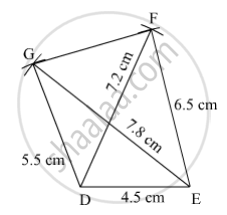SSC (English Medium) Class 8Maharashtra State Board
Share

# Construct the Following Quadrilateral of Given Measures. Construct ☐ Defg Such that L(De) = 4.5 Cm, L(Ef) = 6.5 Cm, L(Dg) = 5.5 Cm, L(Df) = 7.2 Cm, L(Eg) = 7.8 Cm. - SSC (English Medium) Class 8 - Mathematics

#### Question

Construct the following quadrilateral of given measures.

Construct ☐ DEFG such that l(DE) = 4.5 cm, l(EF) = 6.5 cm, l(DG) = 5.5 cm, l(DF) = 7.2 cm, l(EG) = 7.8 cm.

#### Solution

Steps of Construction:

Step 1:
Draw DE = 4.5 cm.

Step 2: With D as centre and radius 7.2 cm, draw an arc.

Step 3: With E as centre and radius 6.5 cm, draw an arc cutting the previous arc at F.

Step 4: Join EF.

Step 5: With D as centre and radius 5.5 cm, draw an arc.

Step 6: With E as centre and radius 7.8 cm, draw an arc cutting the previous arc at G.

Step 7: Join DG and GF.Here, DEFG is the required quadrilateral.

Is there an error in this question or solution?

#### APPEARS IN

Balbharati Solution for Balbharati Class 8 Mathematics (2019 to Current)
Chapter 8: Quadrilateral : Constructions and Types
Practice Set 8.1 | Q: 2 | Page no. 43
Solution Construct the Following Quadrilateral of Given Measures. Construct ☐ Defg Such that L(De) = 4.5 Cm, L(Ef) = 6.5 Cm, L(Dg) = 5.5 Cm, L(Df) = 7.2 Cm, L(Eg) = 7.8 Cm. Concept: Construction of a Quadrilateral.
S